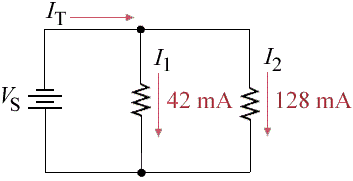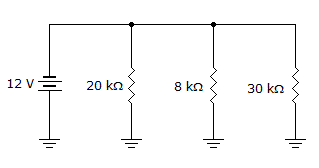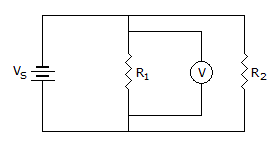# Electronics - Parallel Circuits

Exercise : Parallel Circuits - Filling the Blanks
1.The total current in the given circuit equals _____.

86 mA
170 mA
42 mA
128 mA
Explanation:
No answer description is available. Let's discuss.

2.
As additional resistors are connected to a parallel circuit, the total resistance will ___.
increase
increase by the value of the resistor being connected
decrease
remain unchanged
Explanation:
No answer description is available. Let's discuss.

3.
An ammeter is connected in ______ with the branch resistor to measure branch current, and in ______ with the voltage supply to measure total parallel circuit current.
parallel, series
series, parallel
series, series
parallel, parallel
Explanation:
No answer description is available. Let's discuss.

4.The total current in the given circuit is ___.

2.5 mA
0.6 mA
1.5 mA
0.4 mA
Explanation:
No answer description is available. Let's discuss.

5.If the voltmeter indicates 12 V in the given circuit, the voltage across R2 equals ___.

12 V
VS minus 12 V
0 V